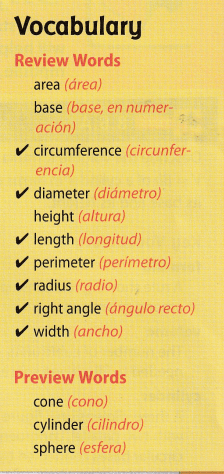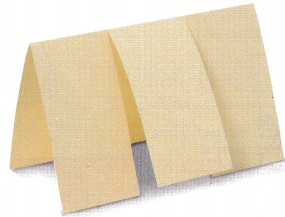Refer to our Texas Go Math Grade 8 Answer Key Pdf to score good marks in the exams. Test yourself by practicing the problems from Texas Go Math Grade 8 Module 9 Answer Key Volume.

Evaluate each exponential expression

Question 1.
112 ___________
Multiply the base (11) by itself the number of times indicated by the exponent (2). Then find the product of all the terms.
112 = 11 × 11 = 121

Question 2.
25 ____________
25 = 2 × 2 × 2 × 2 × 2 = 32

Question 3.
($$\frac{1}{5}$$)3 ___________
($$\frac{1}{5}$$)3 = $$\frac{1}{5}$$ × $$\frac{1}{5}$$ × $$\frac{1}{5}$$ = ($$\frac{1}{125}$$)

Question 4.
(0.3)2 ___________
(0.3)2 = $$\frac{3}{10}$$ × $$\frac{3}{10}$$ = $$\frac{9}{100}$$ = 0.09

Question 5.
2.13 ____________
2.13 = 2.1 × 2.1 × 2.1 = 9.261

Question 6.
0.13 ____________
(0.1)3 = $$\frac{1}{10}$$ × $$\frac{1}{10}$$ × $$\frac{1}{10}$$ = $$\frac{1}{1000}$$ = 0.001

Question 7.
($$\frac{9.6}{3}$$)2 ___________
($$\frac{9.6}{3}$$)2 = (3.2)2 = 3.2 × 3.2 = 10.24

Question 8.
1003 ____________
(100)3 = 100 × 100 × 100 = 1000000

Round to the underlined place.

Question 9.
2.374 _____
2.374 → 2.37

Question 10.
126.399 _____
126.399 → 126

Question 11.
13.9577 _____
13.9577 → 14.0

Question 12.
42.690 _________
42.690 → 42.69

Question 13.
134.95 _____
134.95 → 135.0

Question 14.
2.0486 ______
2.0486 → 2.0

Question 15.
63.6352 _______
63.6352 → 63.64

Question 16.
98.9499 ________
98.9499
The digit to be rounded is 9. The digit to its right is 4, which is less than 5, so the rounded number is 98.9.
98.9499 → 98.9

Simplify each expression.

Question 17.
3.14 (5)2 (10) _______
To simplify the expression, we simplify the exponent and we multiply from left to right.
3.14 ∙ (5)2 ∙ 10 = 3.14 ∙ 25 ∙ 10
= 78.5 ∙ 10
= 785

Question 18.
$$\frac{1}{3}$$(3.14) (3)2 (5)
($$\frac{1}{3}$$) × (3.14) × (3)2 × (5) = 3 × 3.14 × 5 = 47.1

Question 19.
$$\frac{4}{3}$$ (3.14) (3)3 __________
($$\frac{4}{3}$$) × (3.14) × 27 = 4 × 3.14 × 9 = 113.04

Question 20.
$$\frac{4}{3}$$ (3.14) (6)3 _______
($$\frac{4}{3}$$) × (3.14) × (63) = ($$\frac{4}{3}$$) × 3.14 × 216 = 904.32

Question 21.
3.14 (4)2 (9) _______
3.14 × (42) × 9 = 3.14 × 16 × 9 = 452.16

Question 22.
$$\frac{1}{3}$$(3.14) (9)2 ($$\frac{2}{3}$$)
($$\frac{1}{3}$$) × ($$\frac{2}{3}$$) × (92) × 3.14 = ($$\frac{1}{3}$$) × ($$\frac{2}{3}$$) × 81 × 3.14 = 2 × 3.14 × 9 = 56.52

Visualize Vocabulary

Use the ✓ words to complete the empty columns in the chart. You may use words more than once.Understand Vocabulary

Complete the sentences using the preview words.

Question 1.
A three-dimensional figure that has one vertex and one circular base is a __________.
A three-dimensional figure that has one vertex and one circular base is a cone.

Question 2.
A three-dimensional figure with all points the same distance from the center is a __________.